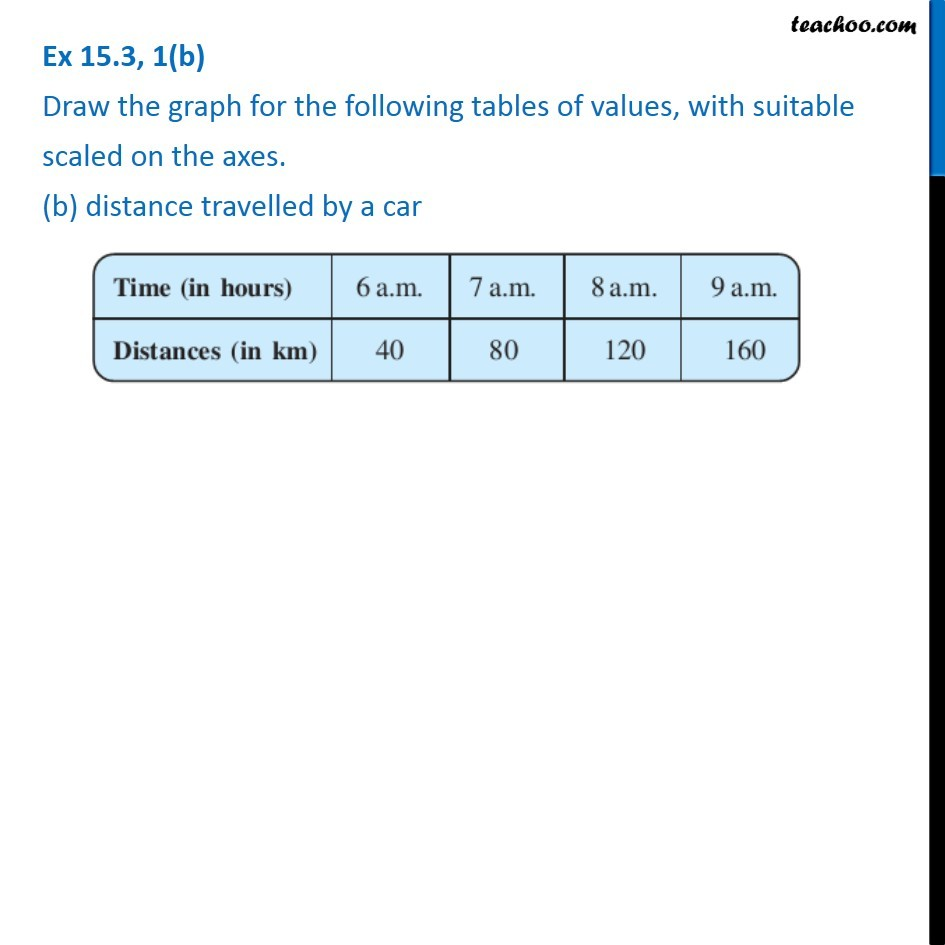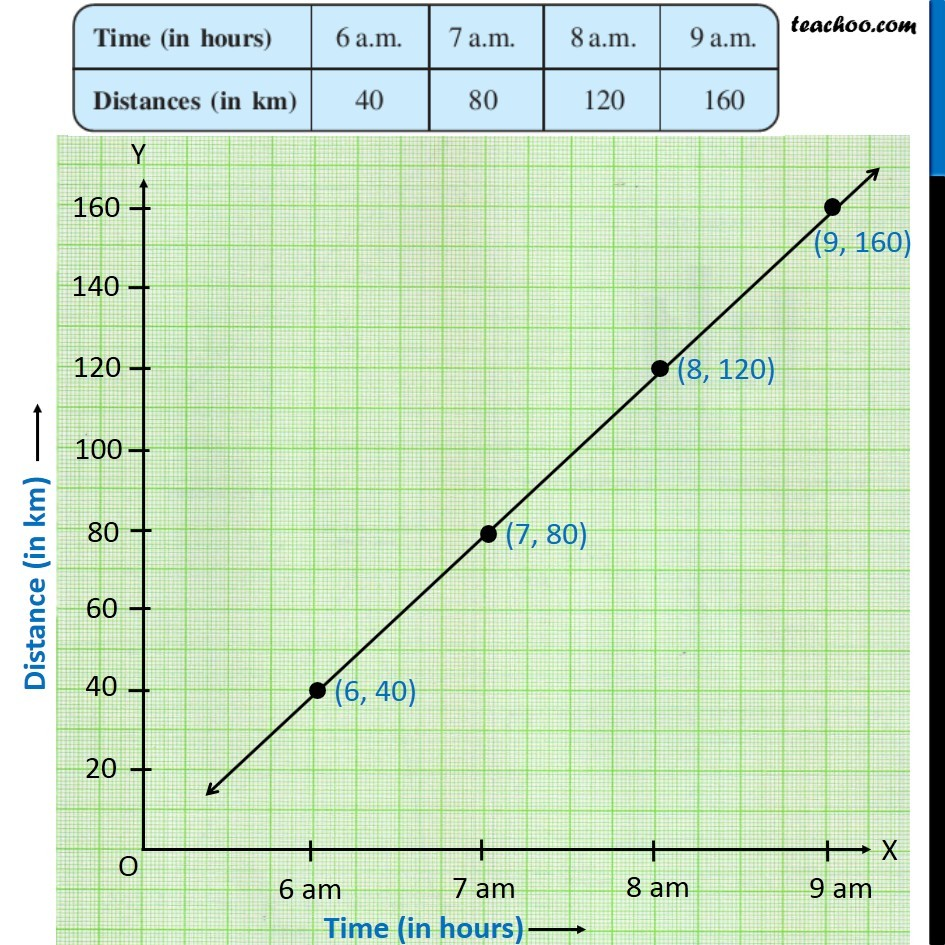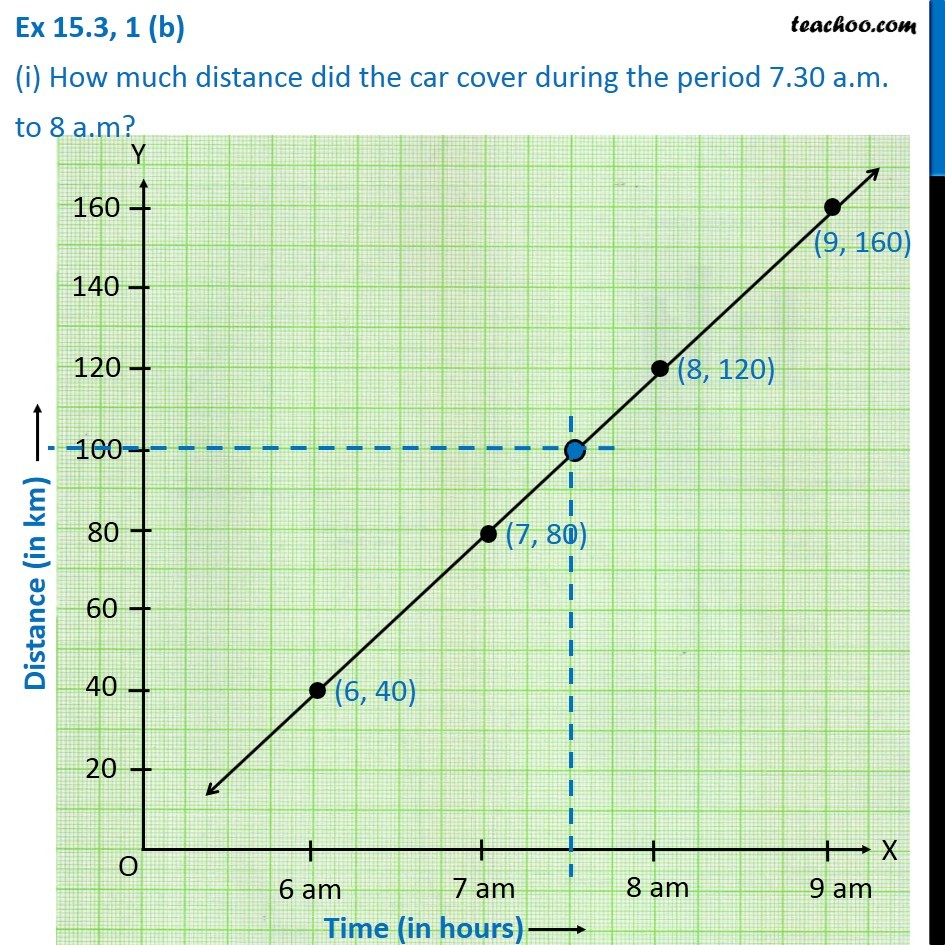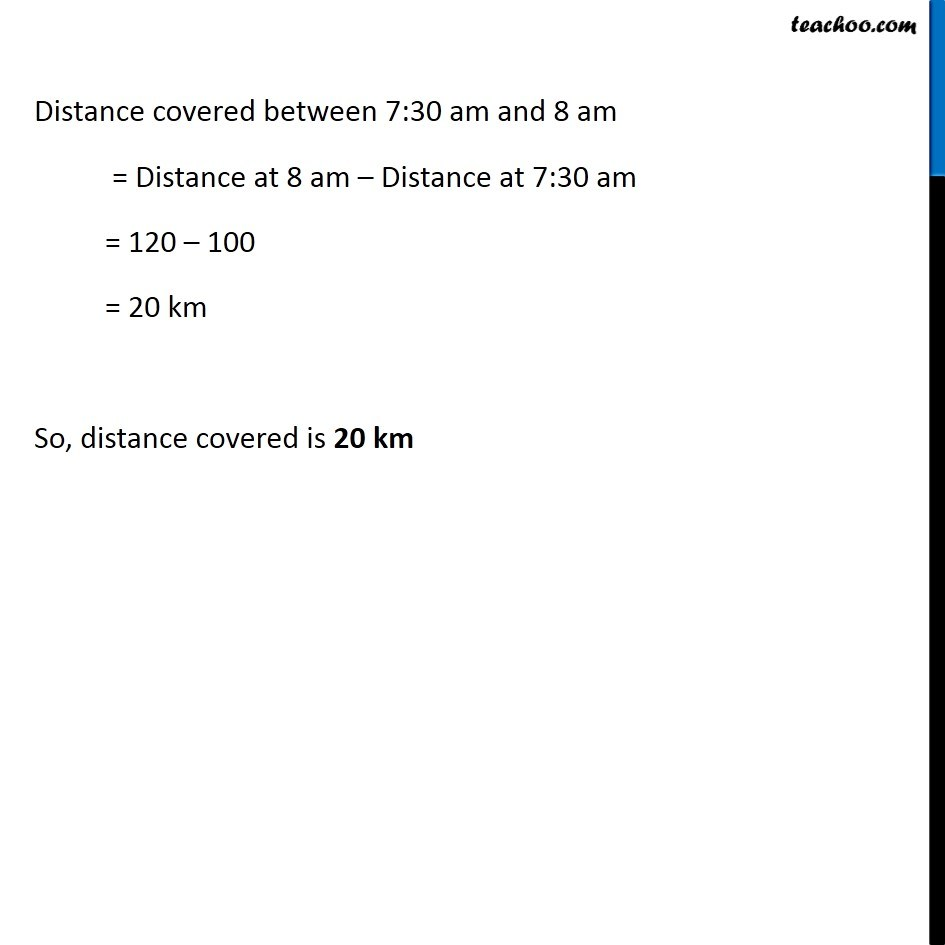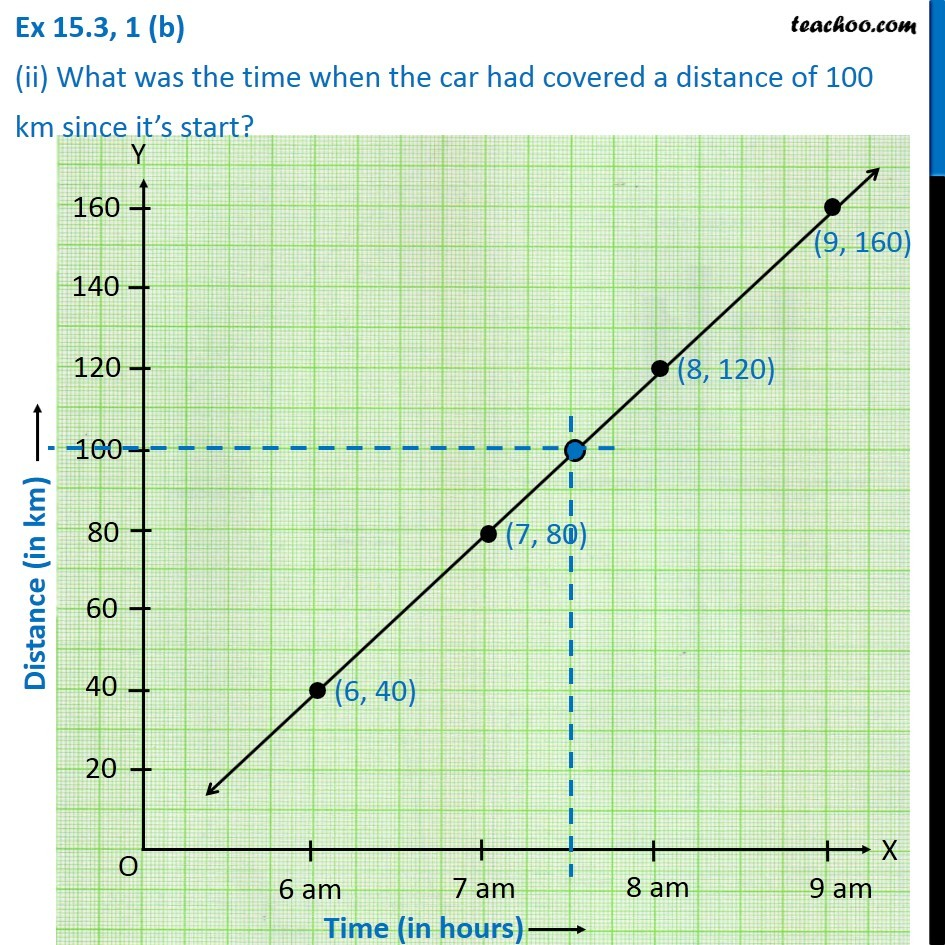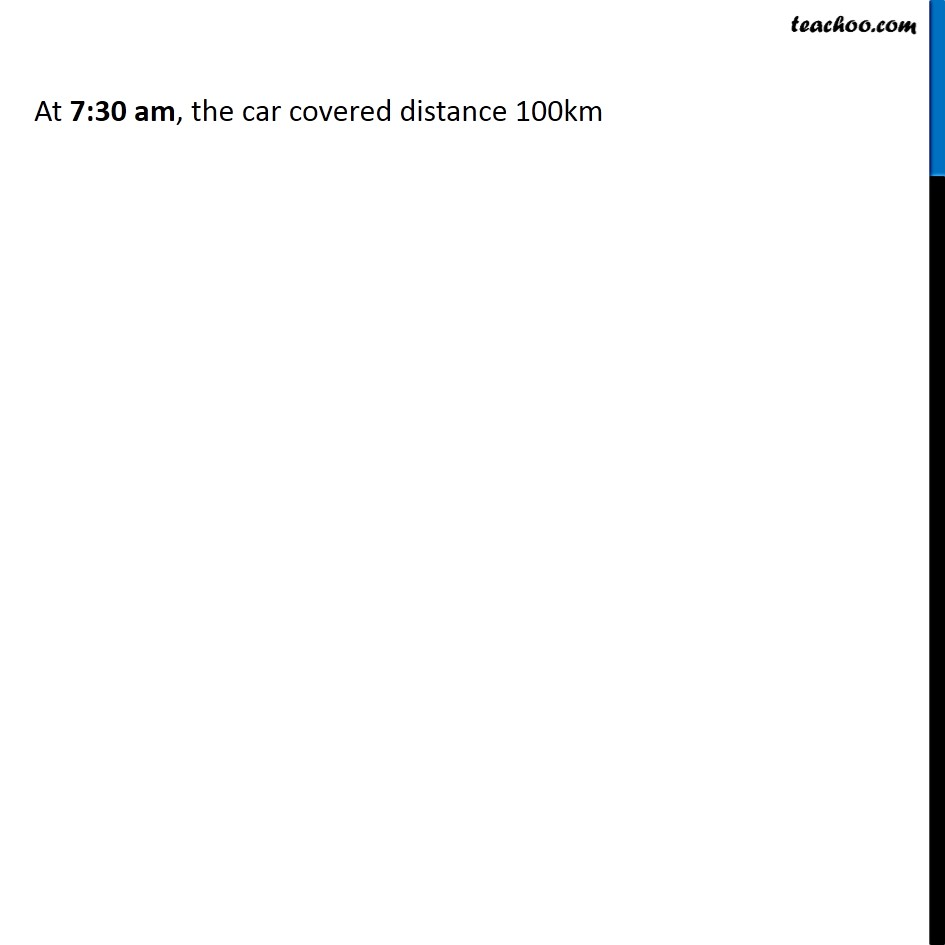Subscribe to our Youtube Channel - https://you.tube/teachoo

1. Chapter 15 Class 8 Introduction to Graphs
2. Concept wise
3. Making Linear Graphs

Transcript

Ex 15.3, 1(b) Draw the graph for the following tables of values, with suitable scaled on the axes. (b) distance travelled by a car Time (in hours) | 6 am | 7 am | 8 am | 9 am Distance (in km) | 40 | 80 | 120 | 160 Plotting Distance (in km), from 0 to 160 (with a gap of 20) and Time (in hours) in y-axis, from 0 to 9 am (with a gap of 5) Ex 15.3, 1 (b) (i) How much distance did the car cover during the period 7.30 a.m. to 8 a.m? Distance covered between 7:30 am and 8 am = Distance at 8 am – Distance at 7:30 am = 120 – 100 = 20 km So, distance covered is 20 km Ex 15.3, 1 (b) (ii) What was the time when the car had covered a distance of 100 km since it’s start? Drawing a vertical line from 7:30 am, Where it intersects the line, draw a horizontal line, distance is 100 km At 7:30 am, the car covered distance 100km

Making Linear Graphs